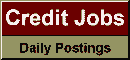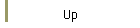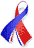DefaultRisk.com the web's biggest credit risk modeling resource.doi> search: A or B Export citation to:- HTML- Text (plain)- BibTeX- RIS- ReDIF

A Comparative Analysis of Hyperbolic Copulas Induced by a One Factor Levy Model

by Henrik Brunlid of Lund University

August 20, 2006

Abstract: In the credit derivatives market, the observed default correlation smile, implied by the Gaussian copula, constitutes a major problem when we want to price bespoke CDO tranches. The industry standard approach for countering this dilemma is to use the concept of base correlation to try to estimate the ingoing default correlation parameters for non-standard tranche intervals. However, this approach is far from mathematically consistent and the method of interpolating between different points on the base correlation curve is all but accurate. In this paper, we generalize the Gaussian one factor model proposed by Vasicek (1987) to work with any underlying Lévy process. Further, we show that our one Factor Lévy model induces an infinite number of different copulas. Given this mathematical framework, we propose three different hyperbolic copulas, namely a normal inverse Gaussian copula, a variance gamma copula and a skewed Student t copula. In addition to calculate the loss distributions by FFT methods, we generalize the LHP approximation given by Vasicek (1991) to work with our Lévy model. Finally, we show that the proposed alternative copulas give superior results and we give a comparative view on the different methods for calculating the loss distributions.

Keywords: hyperbolic distributions, NIG, skewed student t, VG, loss distribution, CDO, Fourier.

Books Referenced in this paper:  (what is this?)